Next: Acknowledgments Up: The ClassAd Language Reference Previous: Syntax

Subsections

# Evaluation

This section defines the semantics of the ClassAd language by explaining how to evaluate an expression.In this section, expression'' means an internal-form expression tree. In general, a composite expression is evaluated by recursively evaluating its component sub-expressions and then using its top-level operator to combine the results. However, there are situations in which evaluation of an expression E depends on parts of a context, which is an expression containing E as a sub-expression. For example, in the expression

    [ a = 3;  b = [ c = a ] ],

the second occurrence of a (an attribute reference) is evaluated by searching the two containing Record expressions for a definition of a, yielding the constant 3.

More formally, an expression in context (EIC) is a pair (E, C) consisting of an expression C (the context) and a designated occurrence of a sub-expression E of C. The semantics of the ClassAd language is defined by a recursive function eval from EICs to EICs. A top-level EIC is an EIC of the form (E, E). For brevity, we will occasionally abbreviate the top-level EIC (E, E) as E, particularly when E is a literal constant. For example, the EIC (error, error) may be written as error. An expression E is evaluated by computing eval(E, E) and extracting the sub-expression from the resulting EIC.

The set of EICs with context C is partially ordered by the relation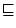, defined by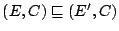iff E is a sub-expression of E'. When we speak of the minimal'' EIC with a given property, we mean the one that is minimal with respect to. An EIC (E, C) is called a scope if the top-level operator of E is RECORD.

Define lookup(s, (E, C)), where s is a string and (E, C) is an EIC, to be the EIC (E', C'), where

• If s matches14the string "parent" and there is a scope (Ep, C) such that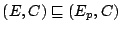, then (E', C') = (Ep, C), where (Ep, C) is the minimal such scope.
• Otherwise, if there is a scope (Ed, C) such that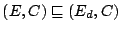and Ed contains a definition t = Et such that t matches s, let t = Et be the definition in the minimal such scope. Then (E', C') = (Et, C).
• Otherwise, (E', C') = (undefined, undefined).

For example, let C be the expression

    [ a = x;  b = [ a = y; c = a]; d = a ],

and let R denote the inner Record expression. C contains two occurrences of the attribute-reference expression a. Let E1 denote the occurrence inside R and E2 the other occurrence. Then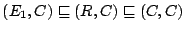,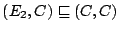,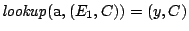,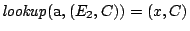,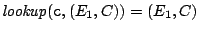, and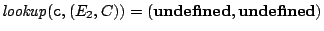.

## Types, Undefined, and Error

Each expression has a type, which is one of Integer, Real, String, Boolean, AbsTime, RelTime, Undefined, Error, List, or Record. The types Integer and Real are collectively called numeric types. The types AbsTime and RelTime are collectively called timestamp types. Each operator imposes constraints on the types of its operands. If these constraints are not met, the value returned by the operator is error.

An attribute reference with attribute name N evaluates to undefined if the reference is not contained in any scope that defines N. It may also evaluate to undefined in the presence of loops, as in

    [ a = b; b = a ].


Most operators are strict'' with respect to undefined and error. The only exceptions are the Boolean operators described in Section 4.3.1, the operators is and isnt described in Section 4.3.2, and the LIST and RECORD constructors described in Section 4.3.8. Strict evaluation obeys the following ordered sequence of rules.

• If the operands do not obey the type restrictions imposed by the operator, the result of the evaluation is error. The following sections list all combinations of types accepted by each operator. None of the strict operators accept operands of type Error, so this rule implies that if any sub-expression evaluates to error, the expression evaluates to error. This rule also catches type errors'' such as "foo" / 3.
• Otherwise, if any operand of a strict operator is undefined, the result is undefined.
• Otherwise, the result is computed from the operands as described in the following sections.

## Atomic Expressions

A literal constant evaluates to itself. More precisely, if c is an occurrence of a literal constant, then eval(c, C) = (c, C).

If x is an attribute reference with attribute name N, then eval(x, C) = eval(lookup(N, (x, C))). In particular, (x, C) evaluates to undefined if there is no scope (R, C) containing the indicated occurrence of x such that R defines N. If this recursive definition leads directly or indirectly to a call eval(x, C), the result is undefined.

## Composite Expressions

List and Record expressions evaluate to themselves. More precisely, if E is an expression whose root operator is LIST or RECORD, eval(E, C) = (E, C). The operators SELECT and SUBSCRIPT are discussed below. For all other operators, evaluation is bottom-up'' and the result is a pure value''. More precisely, ifis a binary operator other than SELECT, or SUBSCRIPT, then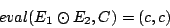where

eval(E1, C) = (E1', C1),

eval(E2, C) = (E2', C2),

and c is the (literal constant) result of applying operatorto the expressions E1' and E2', as defined in the following sections. Similar rules apply to unary and ternary operators.

The operators found in C, C++, or Java are generally evaluated according to the rules of those languages. In cases where the specifications of those languages differ, the ClassAd language follows the Java semantics because it is more precise (the C and C++ specifications occasionally say the results are undefined'' or implementation defined'' in unusual situations). The only deviations from Java semantics involve exceptions. In cases where Java specifies that evaluation throws an exception, the ClassAd language returns the constant error. The constants error and undefined also require special treatment when supplied as arguments to operators.

### Boolean Operators

The Boolean operators && and || and the ternary operator _?_:_ are evaluated left to right'' with respect to error, and optimistically'' with respect to undefined. For example,

true || x = true
false && x = false
undefined || true = true
true ? val : x = val
false ? x : val = val

even if x evaluates to error or undefined.

The Boolean operators treat Boolean true, false, and undefined as a three-element lattice with

false < undefined < true.
With respect to this lattice, && returns the minimum of its operands, || returns the maximum, and ! interchanges true and false.

The complete definition of the operators &&, &&, !, and _?_:_ is given by the tables

          && | F U T O    || | F U T O    ! |      ?:|
---+--------    ---+--------    --+--   ---+---
F | F F F F     F | F U T E    F | T    F | expr3
U | F U U E     U | U U T E    U | U    U |  U
T | F U T E     T | T T T T    T | F    T | expr2
O | E E E E     O | E E E E    O | E    O |  E

In these tables, the letters T, F, U, and E stand for the constants true, false, undefined, and error, respectively; O stands for any expression other than true, false, or undefined (including error); and expr2 and expr3 represent the second and third operands of the expression expr1 ? expr2 : expr3.

### is and isnt

The expression expr1 is expr2 evaluates to true if expr1 and expr2 evaluate to identical'' values and false otherwise. The expression expr1 isnt expr2 evaluates to the negation of expr1 is expr2. These operators are most commonly used to test for undefined or error as in
    result = (expr is undefined) ? 0 : (expr + 1);

but they can be used to compare arbitrary values.

For the purposes of this section, the relationship identical'' is defined as follows.

• Expressions of different types are never identical. Thus (3 is 3.0) (3 is "3") and (undefined is error) all evaluate to false.
• Two values of type Integer, Real, or RelTime are identical if and only if they are numerically equal constants.
• Two values of type AbsTime are identical if they represent the same instant in time and the same offset from UTC.
• Two values of type Boolean are identical if and only if they are both true or both false.
• Two values of type Undefined or two values of type Error are identical. Thus ((3 * "x") is error) evaluates to true.
• Two values of type String are identical if and only if they are identical character by character. Case is significant. Thus ("One" == "one") and ("One" isnt "one") both evaluate to true.
• Two values of type List or Record are identical if and only if they are created by the same instance of a LIST or RECORD constructor operator. For example, if R is the expression
        [ a = { 1, 2 }; b = { 1, 2 }; c = a is b; d = a is a ],

then R.c evaluates to false, while R.d evaluates to true.
Note that the is and isnt operators always evaluate to true or false, never undefined or error.

### Comparison Operators

For the six comparison operators <, <=, ==, !=, >=, and >, both operands must be numeric (Integer or Real), both String, both AbsTime, or both RelTime. Otherwise, the result is error. If one operand is Integer and the other is Real, the Integer argument is first converted to Real. The results are calculated as in Java .

If the operands are Strings, they are converted to lower case and compared lexicographically.

If the operands are AbsTimes, they are equal if they correspond to the same instant (according to UTC). Otherwise, the earlier time is less than the later one. If the operands are RelTimes, they are compared as signed integers.

### Arithmetic Operators

The unary operators +, -, and binary operators +, -, *, /, %, take numeric operands.15The results are calculated as in Java ,16with one exception: Integer division or remainder when the second operand is zero throws an ArithmeticException in Java, but returns error in the ClassAd language. In particular, if operands are Integers, the result is an Integer, and if one operand of a binary operation is an Integer and the other is a Real, the Integer operand is converted to a Real and the result is computed using 64-bit floating point arithmetic. The integral / operation truncates the result towards zero, and the integral % operation generally returns a result with the same sign as the dividend (the left operand). See the Java language specification  for details.

The unary and binary operators + and - are also defined for certain timestamp operands. The unary + operator is applicable to both AbsTime and RelTime operands and returns the value of its operand unchanged. The unary - operator is applicable only to RelTime operands and returns the RelTime value with the same magnitude and opposite sign.

The rules for binary operators are summarized in Table 6. If the result of an expression is an AbsTime, its time zone is the same as the time zone of the AbsTime argument.

Table 6: Date and Time Arithmetic
 Expression Result type Result value AbsTime + AbsTime error AbsTime + RelTime AbsTime The AbsTime operand offset by the amount of the RelTime operand RelTime + AbsTime AbsTime The AbsTime operand offset by the amount of the RelTime operand RelTime + RelTime RelTime The numeric sum of the two operands AbsTime - AbsTime RelTime The numeric difference of the two operands AbsTime - RelTime AbsTime The AbsTime operand offset by the negative of the RelTime operand RelTime - AbsTime error RelTime - RelTime RelTime The numeric difference of the two operands

### Bitwise Boolean Operators

The bitwise logical unary operator ~ and binary operators |, ^, and & are defined only for Integer and Boolean operands. They are defined to return the same results as the corresponding operators in Java .

### Shift Operators

The shift operators << (left shift), >> (right shift with sign extension), and >>> (right shift with zero fill) are defined only for Integer operands.17They are defined to return the same results as the corresponding operators in Java .

### Select and Subscript

The SELECT operator has two operands, the base and the selector, where the selector is syntactically constrained to be an attribute name. In the native syntax, it is written base.selector. It is semantically equivalent to base["selector"]. That is, an instance of SUBSCRIPT operator where the subscript is the string value corresponding to the attribute name. For example,
    [ rec = [ One = 1; Two = 2 ]; val = rec.one ].val

and
    [ rec = [ One = 1; Two = 2 ]; val = rec["one"] ].val

both evaluate to 1. The SELECT syntax is more concise, but the SUBSCRIPT syntax is more flexible, because it allows the selector to be computed rather than requiring a literal string.

The SUBSCRIPT operator has two operands, the base and the subscript. In the native syntax, it is written base[subscript]. The subscript expression must have type Integer or String. If the subscript is an Integer i, the base expression must have type List and the result is the ith element of the list, counting from zero. If the subscript is a String s, the base expression must be a Record or List. If the base expression has type Record, the result is computed by searching the base and its containing scopes for an attribute definition matching the attribute name s. If the base expression is a List, the SUBSCRIPT operator is applied to each member of the list and the result is a new top-level'' list of the results. In all other cases, the result is error.

More precisely,

eval(Eb[Es], C) = (E', C'),

where E' and C' are defined as follows. Let

eval(Eb, C) = (Eb', Cb')

and

eval(Es, C) = (Es', Cs').

• If Es' is an Integer with value i and Eb' is a list { E0, ..., En - 1} with n > i members, then (E', C') = (Ei, Cb').
• If Es' is a String with value s and Eb' is a Record expression, then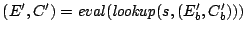.
• If C2 is a String with value s and Eb' is a list { E0, ..., En - 1}, then E' is the list { E0', ..., En - 1'}, where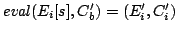and C' = E' (that is, the result is a top-level'' EIC). Note that the environments Ci' returned by the recursive calls to eval are discarded.
• In all other cases, the result is error.

### List and Record Constructors

The LIST operator takes as operands an arbitrary sequence of values of arbitrary types. The RECORD operator takes as operands a sequence of definitions of the form namei = valuei, where the valuei are arbitrary values. The result is the Record
[ name0 = value0; ... ; namen-1 = valuen-1]

List and Record expressions evaluate to themselves. That is, eval(E, C) = (E, C) if E is of type List or Record.

### Function Calls

The FUNC_CALL operator takes a function name and zero or more operands. Function names are matched regardless of case, so that substr("abc",2), SubStr("abc",2), and SUBSTR("abc",2) all invoke the same function.

Currently, all functions are strict with respect to error and undefined, unless otherwise specified. In other words, if any argument evaluates to error or undefined, the result is error or undefined, respectively. If arguments of both types are present, the result is error.

Currently, all functions return top-level'' values that are independent of the the context of the call. That is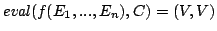, where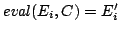for i = 1,...,n and V is a value computed from E1', ..., En' as described in the following table.

The following table lists all functions required by the current version of this specification; others may be added in future versions. The description of each function is preceded by a prototype indicating restrictions on the number and types of arguments and indicating the type of the result returned. If the restrictions are violated, the result is error. In the prototypes, const'' stands for any literal constant of type Integer, Real, String, Boolean, AbsTime, or RelTime (but not Undefined, Error, List, or Record), and any'' means any expression. A type followed by an asterisk indicates any number of arguments of the indicated type, including none. Square brackets are used to indicate optional arguments.

isUndefined(any a) returns boolean.
Returns true is a is the undefined value, otherwise returns false. This function is not strict.

isError(any a) returns boolean.
Returns true is a is the error value, otherwise returns false. This function is not strict.

isString(any a) returns boolean.
Returns true is a is a string value, otherwise returns false. This function is not strict.

isInteger(any a) returns boolean.
Returns true is a is an integer value, otherwise returns false. This function is not strict.

isReal(any a) returns boolean.
Returns true is a is a real value, otherwise returns false. This function is not strict.

isList(any a) returns boolean.
Returns true is a is a list value, otherwise returns false. This function is not strict.

Returns true is a is a record value, otherwise returns false. This function is not strict.

isBoolean(any a) returns boolean.
Returns true is a is a boolean value, otherwise returns false. This function is not strict.

isAbstime(any a) returns boolean.
Returns true is a is an AbsTime value, otherwise returns false. This function is not strict.

isReltime(any a) returns boolean.
Returns true is a is a RelTime value, otherwise returns false. This function is not strict.

int(const x) returns int.

The result is x converted to an Integer. If x is an Integer, the result is x. If x is a Real, it is truncated (towards zero) to an integer. If x is true the result is 1. If x is false the result is 0. If x is an AbsTime, it it converted to the number of seconds since the epoch, UTC. If x is a RelTime, it it converted to a number of seconds. If x is a String, it is parsed according to the native syntax for integer_literal or floating_point_literal as in Table 4 and then converted to an Integer as above. If x is a String that does not represent a valid integer or floating-point literal, the result is error.

real(const x) returns real.

The result is x converted to a Real. If x is a Real, the result is x. If x is an Integer, it is converted to Real. If x is true the result is 1.0. If x is false the result is 0.0. If x is an AbsTime, it it converted to the number of seconds since the epoch, UTC. If x is a RelTime, it it converted to a number of seconds. If x is a String, it is parsed according to the native syntax for integer_literal or floating_point_literal as in Table 4 and then converted to a Real as above. In addition, the strings INF, -INF and NaN (in any combination of upper and lower case) are recognized as representing the IEEE754 values for positive and negative infinity and not-a-number, respectively. If x is a String that does not represent a valid integer or floating-point literal, the result is error. For any other type, x is converted to an Integer as if by int'', and the result is converted to a Real (or error if the conversion to Integer fails).

string(any x) returns string.

If x is a String, the result is x. Otherwise, the result is the canonical unparsing of x (see Section 3.3.3).

floor(const x) returns int.

If x is an Integer, the result is x. Otherwise, x is converted to a real by the function real'' above, and the result is the largest integer not greater than that value (or error if the conversion fails).

ceiling(const x) returns int.

If x is an integer, the result is x. Otherwise, x is converted to a real by the function real'' above, and the result is the smallest integer not less than that value (or error if the conversion fails).

round(const x) returns int.

If x is an integer, the result is x. Otherwise, x is converted to a real y by the function real'' above, and the result is the nearest integer to y. If y is midway between two integers, the even integer is returned. The result is error if the conversion fails or the resulting integer does not fit in 32 bits.

random(number x) returns int.

If x is an integer, the result is an integer random number r in the range 0 <= r < x. If x is a real number, the result is a real random number in the same range.If x is anything else, the result is an error.

strcat(any*) returns string.

Each argument is converted to a string by the function string'' above. The result is the concatenation of the strings.

substr(string s, int offset [, int length ]) returns string.
The result is the substring of s starting at the position indicated by offset with the length indicated by length. The first character of s is at offset 0. If offset is negative, it is replaced by length(s) - offset. If length is omitted, the substring extends to the end of s. If length is negative, an intermediate result is computed as if length were omitted, and then -length characters are deleted from the right end of the result. If the resulting substring lies partially outside the limits of s, the part that lies withing s is returned. If the substring lies entirely outside s or has negative length (because of a negative length argument), the result is the null string. [Note: This function is the same as the substr function of Perl.]

strcmp(any a, any b) returns int.
The operands are converted to strings by the string'' function above. The result is an integer less than, equal to, or greater than zero according to whether a is lexicographically less than, equal to, or greater than b. Note that case is significant in the comparison.

stricmp(any a, any b) returns int.

The same as strcmp except that upper and lower case letters are considered equivalent.

toUpper(string s) returns string.

The operands are converted to strings by the string'' function above. The result is a string that is identical to s except that all lowercase letters in s will be converted to uppercase.

toLower(string s) returns string.

The operands are converted to strings by the string'' function above. The result is a string that is identical to s except that all uppercase letters in s will be converted to lowercase.

member(const x, string l) returns boolean.

If x is not a constant or l is not a list, then the result is an error. Otherwise, if any of the elements is equal to x in the sense of the == operator, then the result is true, otherwise it is false.

regexp(string pattern, string target, string options) returns boolean.

If the regular expression pattern matches the target, this function returns true, but otherwise returns false.

Unfortunately, the allowed patterns and options cannot be precisely defined at this time because different ClassAd implementations use different underlying libraries to implement regular expression matching. The Java implementation uses Sun's regular expression implementation, which is Perl-like. The C++ implementation uses either POSIX or Perl-compatible regular expressions, depending on what is available at compilation time. This dichotomy is unfortunate, and we hope to resolve it in the future. For now, you must either know details about your ClassAd implementation, or you must use a subset of regular expressions that are POSIX- and Perl- compatible.

The options are specified as a string of letters in any order. Each letter indicates a single option. For POSIX regular expression matching, the only option is i'' for case-insensitive matching. For Perl-compatible regular expressions, there are four options: i'' for case-insensitive matching, m'' for multiline matching, x'' for extended syntax, and s'' to cause the dot character (.) to match all characters, including newlines.

identicalMember(const x, string l) returns boolean.

If x is not a constant or l is not a list, then the result is an error. Otherwise, if any of the elements is equal to x in the sense of the is operator, then the result is true, otherwise it is false.

time() returns int.

Returns the current Coordinated Universal Time, in seconds since midnight January 1, 1970.

interval(int t) returns string.

The operand t is treated as a number of seconds. The result is a string of the form days+hh:mm:ss. Leading components are omitted if they are zero. For example, if the operand is 1472523 = 17*24*60*60 + 1*60*60 + 2*60 + 3 (seventeen days, one hour, two minutes, and three seconds), the result is "17+1:02:03"; if the operand is 67, the result is "1:07".

absTime(string s) returns AbsTime.

The operand s is parsed as a specification of an instant in time (date and time). This function accepts the canonical native representation of AbsTime values, but minor variations in format are allowed.

The default format is yyyy-mm-ddThh:mm:sszzzzz where zzzzz is a time zone in the format +hh:mm or -hh:mm, but variations are allowed.

• Each separator character -, :, or T may be omitted or replaced by any sequence of non-digits. Note, however, that the - in a time zone of the form -hh:mm may not be omitted.
• The colon between hh and mm in the time zone may be omitted.
• An arbitrary sequence of non-digit characters may precede zzzzz or yyyy.
• The zone may be replaced by the character z or Z, which is equivalent to -00:00.
• The zone may be omitted, in which case the local time zone is used. If the string ends with +dddd, -dddd, z, or Z, where each d is a digit, this suffix is considered to be the time zone indication. For example, in 2003+1030, the suffix 1030 is interpreted as a time zone 10 hours and 30 minutes east, rather than as October 30.
• The fields ss, mm, hh, etc. may be omitted (from right to left), in which case the omitted fields are assumed to be zero.
More precisely, the string must match the regular expression
    D* dddd [D* dd [D* dd [D* dd [D* dd [D* dd D*]]]]] [-dd[:]dd|+dd[:]dd|z|Z]

Where d stands for a digit and D stands for a non-digit.

For example, in the United States central time zone, an AbsTime corresponding to 9 am Jan 25, 2003 CST'' may be created by any of the function calls

 2003-01-25T09:00:00-06:00 // canonical 2003-01-25 09:00:00 -0600 // different separators 20030125090000-0600 // compact format 2003-01-25 16:00:00 +01:00 // different time zone 2003-01-25 15:00Z // omitted seconds, UTC time zone 2003-01-25 09:00:00 // default time zone (local) 2003-01-25 09 // omitted minutes and seconds

and AbsTimes corresponding to Jan 25, 2003'' (implicitly midnight, UTC) may be written

 2003-01-24T18:00:00-06:00 // canonical 2003-01-25T00:00:00 // default time zone: UTC 2003-01-25 // omitted time of day 2003/01/25 // different separators 20030125 // compact format

The strings 2003-01-25T09:00:00-06:00 and 2003-01-25 15:00Z represent the same instant in time, but measured in different time zones.

The following strings are invalid.

 2003-01-25T09:00:00-06 // incomplete time zone 2003-01-25T09:00:00- 0600 // space in time zone 2003-1-25 // missing digit in dd field

absTime([const t[, int z]
) returns AbsTime.]

Creates an AbsTime value corresponding to time t an time-zone offset z. If t is a String, then z must be omitted, and t is parsed as a specification as described above. If t and z are both omitted, the result is an AbsTime value representing the time and place where the function call is evaluated. Otherwise, t is converted to a real by the function real'' above, and treated as a number of seconds from the epoch, Midnight January 1, 1970 UTC. If z is specified, it is treated as a number of seconds east of Greenwich. Otherwise, the offset is calculated from t according to the local rules for the place where the function is evaluated.

relTime(const t) returns RelTime.

If the operand t is a String, it is parsed as a specification of a time interval. This function accepts the canonical native representation of RelTime values, but minor variations in format are allowed.

Otherwise, t is converted to a real by the function real'' above, and treated as a number of seconds.

The default string format is [-]days+hh:mm:ss.fff, where leading components and the fraction .fff are omitted if they are zero. In the default syntax, days is a sequence of digits starting with a non-zero digit, hh, mm, and ss are strings of exactly two digits (padded on the left with zeros if necessary) with values less than 24, 60, and 60, respectively and fff is a string of exactly three digits. In the relaxed syntax,

• Whitespace may be added anywhere except inside the numeric fields days, hh, etc.
• Numeric fields may have any number of digits and any non-negative value.
• The + may be replaced by d or D.
• The first : may be replaced by h or H.
• The second : may be replaced by m or M.
• The letter s or S may follow the last numeric field.
• If field i is terminated with one of the letters dDhHmMsS and the value of field i-1 is zero, field i-1, together with its terminating field name (+, :, h, etc.) may be omitted even if field i-2 is not omitted.
• The fraction .fff may have any number of digits. If it has no digits, the preceding decimal point may be omitted.

For example, one day, two minutes and three milliseconds may have any of the forms

 1+00:02:00.003 // the result of relTimeToString 1d0h2m0.003s // similar to ISO 8601 1d 2m 0.003s // add spaces, omit hours field 1d 00:02:00.003 // mixed representations 1d 00:00:120.003 // number of seconds greater than 59 86520.002991 // seconds, excess precision in fraction

Creates a ClassAd with each component of the time as an element of the ClassAd. The ClassAd has five attributes:

 Type // RelativeTime'' Days // the number of days Hours // the number hours Minutes // the number of minutes Seconds // the number of seconds

Creates a ClassAd with each component of the time as an element of the ClassAd. The ClassAd has five attributes:

 Type // AbsoluteTime'' Year // the year Month // the month, from 1 (January) through 12 (December) Day // the day, from 1 through 31 Hours // the number of hours Minutes // the number of minutes Seconds // the number of seconds Offset // the timezone offset in seconds

formatTime(AbsTime t[, string s]) returns string.

This function creates a formatted string that is a representation of the absolute time t.

The string is similar to the ANSI C strftime function. It consists of arbitary text plus placeholders for elements of the time. These placeholders are percent signs (%) followed by a single letter. To have a percent sign in your output, you must use a double percent sign (%%).

Because an implementation may use strftime() to implement this, and some versions implement extra, non-ANSI C options, the exact options available to an implementation may vary. An implementation is only required to implement the ANSI C options, which are:

 %a // abbreviated weekday name %A // full weekday name %b // abbreviated month name %B // full month name %c // local date and time representation %d // day of the month (01-31) %H // hour in the 24-hour clock (0-23) %I // hour in the 12-hour clock (01-12) %j // day of the year (001-366) %m // month (01-12) %M // minute (00-59) %p // local equivalent of AM or PM %S // second (00-59) %U // week number of the year (Sunday as first day of week) (00-53) %w // weekday (0-6, Sunday is 0) %W // week number of the year (Monday as first day of week) (00-53) %x // local date representation %X // local time representation %y // year without century (00-99) %Y // year with century %Z // time zone name, if any % // %

Note that names may be locale-dependent, if the underlying operating system supports locales. Also note that some ClassAd implementations may have difficulty with time zone names for non-local time zones, since the names may vary.

formatTime(int i[, string s]) returns string.

This version of formatTime converts i to an absolute time, then behaves identically to the other version of formatTime.

#### Footnotes

... matches14
We are using the term match'' here as defined in Section 3.1: Two strings match if they are identical except for differences in case.
... operands.15
Unlike Java, the + operator is not overloaded to accept String operands.
...JLS,16
The % operator is defined for floating point operands according to the Java programming language specification, not according C. Some C implementations may not support % with floating point operands, so users concerned with portability should avoid this special case until all implementations are brought into compliance.
... operands.17
Note that C and C++ have no »> operator. These languages perform a similar operation when the operands are declared to be unsigned. There are no unsigned types in Java or the ClassAd language.Next: Acknowledgments Up: The ClassAd Language Reference Previous: Syntax
Alain Roy 2004-09-30